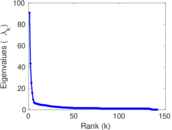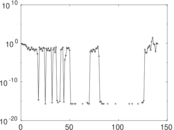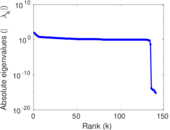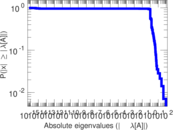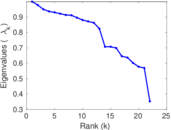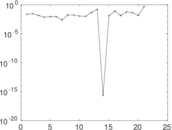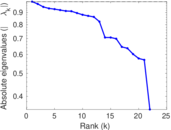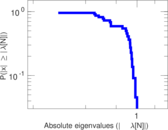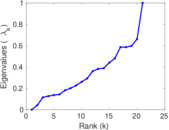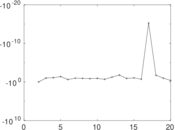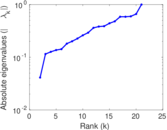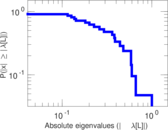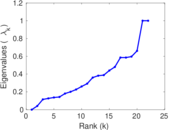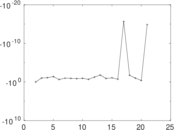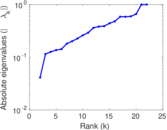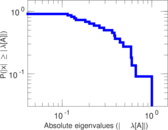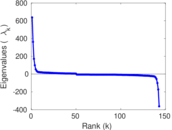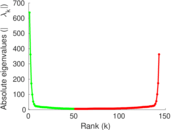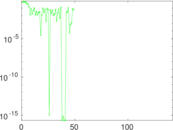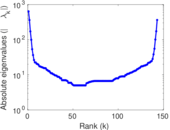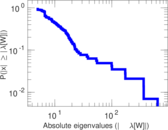# Wikibooks edits (ky)

This is the bipartite edit network of the Kyrgyz Wikibooks. It contains users and pages from the Kyrgyz Wikibooks, connected by edit events. Each edge represents an edit. The dataset includes the timestamp of each edit.

 Code `bky` Internal name `edit-kywikibooks` Name Wikibooks edits (ky) Data source http://dumps.wikimedia.org/ AvailabilityDataset is available for download Consistency checkDataset passed all tests Category Authorship network Dataset timestamp 2017-10-20 Node meaning User, article Edge meaning Edit Network formatBipartite, undirected Edge typeUnweighted, multiple edges Temporal dataEdges are annotated with timestamps

## Statistics

 Size n = 647 Left size n1 = 143 Right size n2 = 504 Volume m = 905 Unique edge count m̿ = 579 Wedge count s = 18,449 Claw count z = 1,030,272 Cross count x = 46,267,843 Square count q = 123 4-Tour count T4 = 76,082 Maximum degree dmax = 201 Maximum left degree d1max = 201 Maximum right degree d2max = 126 Average degree d = 2.797 53 Average left degree d1 = 6.328 67 Average right degree d2 = 1.795 63 Fill p = 0.008 033 63 Average edge multiplicity m̃ = 1.563 04 Size of LCC N = 258 Diameter δ = 8 50-Percentile effective diameter δ0.5 = 2.377 46 90-Percentile effective diameter δ0.9 = 4.332 23 Median distance δM = 3 Mean distance δm = 3.166 27 Gini coefficient G = 0.622 818 Balanced inequality ratio P = 0.262 431 Left balanced inequality ratio P1 = 0.202 210 Right balanced inequality ratio P2 = 0.351 381 Relative edge distribution entropy Her = 0.862 448 Power law exponent γ = 5.591 71 Tail power law exponent γt = 2.741 00 Tail power law exponent with p γ3 = 2.741 00 p-value p = 0.027 000 0 Left tail power law exponent with p γ3,1 = 2.211 00 Left p-value p1 = 0.485 000 Right tail power law exponent with p γ3,2 = 3.491 00 Right p-value p2 = 0.012 000 0 Degree assortativity ρ = −0.153 354 Degree assortativity p-value pρ = 0.000 212 209 Spectral norm α = 90.820 6 Algebraic connectivity a = 0.041 162 5 Spectral separation |λ1[A] / λ2[A]| = 2.102 46 Controllability C = 367 Relative controllability Cr = 0.576 138

## Plots

### Fruchterman–Reingold graph drawing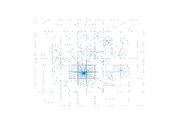### Degree distribution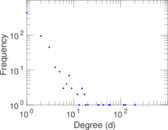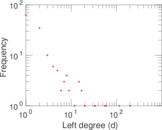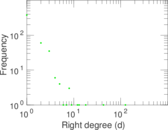### Cumulative degree distribution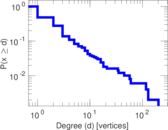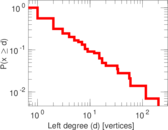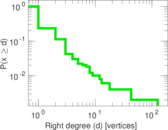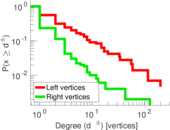### Lorenz curve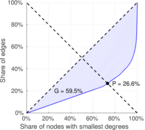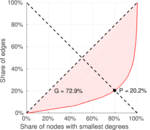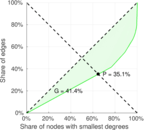### Spectral distribution of the adjacency matrix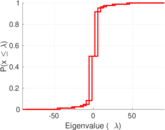### Spectral distribution of the normalized adjacency matrix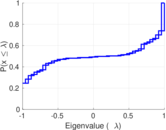### Spectral distribution of the Laplacian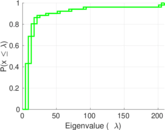### Spectral graph drawing based on the adjacency matrix### Spectral graph drawing based on the Laplacian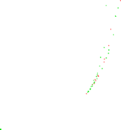### Spectral graph drawing based on the normalized adjacency matrix### Degree assortativity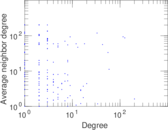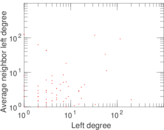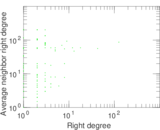### Zipf plot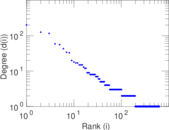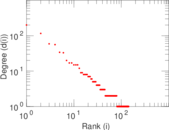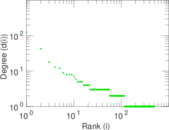### Hop distribution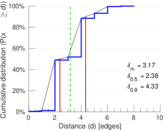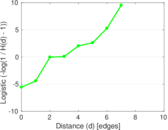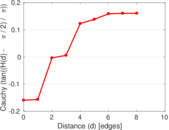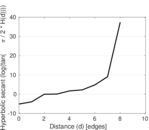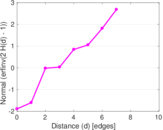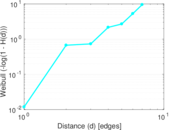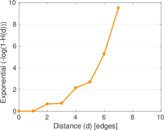### Double Laplacian graph drawing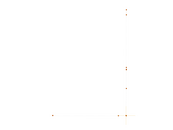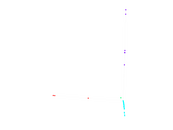### Delaunay graph drawing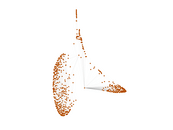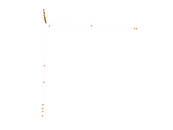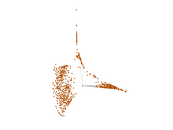### Edge weight/multiplicity distribution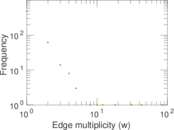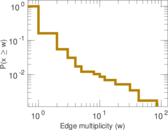### Temporal distribution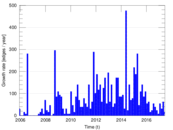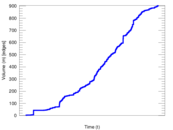### Temporal hop distribution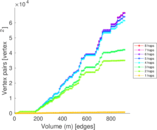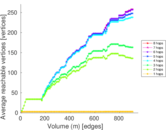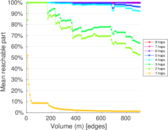### Diameter/density evolution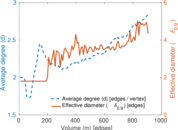### Matrix decompositions plots